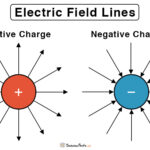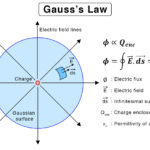Spring Force: Definition, Formula, and Examples
Home / Physics / Spring Force

# SpringForce

## What is SpringForce

When a metal spring is stretched or compressed, it is displaced from its equilibrium position. As a result, it experiences a restoring force that tends to retract the spring back to its original position. This force is called the spring force. It is a contact force that can be found in elastic materials.

## Examples of SpringForce

Here are a few examples of the spring force from everyday life.

• Pendulum
• Rubber band
• Bungee cord
• Keys in a PC keyboard
• Trampoline
• Brakes, clutches, and shock absorbers
• Watches and toys
• Pogo stick
• Spring balance

## SpringForce Equation: How to Find the SpringForce

The spring force can be quantified using Hooke’s law. According to this law, when a spring stresses or compresses, the restoring force F is proportional to the displacement x.

F x

Spring Force Formula:

F = -kx

Unit of Spring Force: Newton or N

Here, k is known as the force constant. The negative sign is because the force is opposite to the displacement, that is, the force displaces the spring from its equilibrium position. This equation applies to both compression and extension of spring. The force can be measured using a spring tester.

The magnitude of the spring constant is given by,

k = F/x

Suppose F = 1 N and x = 1 m, then

k = 1 N/ 1 m = 1 N/m

Therefore, the spring constant is defined as the force required to displace the spring by one meter. It has a unit of Newton per meter (N/m) and a dimension given by MT-2.

Work Done by Spring Force

The work done W by the spring force is given by,

W = F.x

Spring Force on an Object Suspended by a Spring

If an object of mass m is suspended by a spring, then it is displaced by its own weight. The spring force is its weight mg

mg = -kx

Or, |k| = mg/x

The above equation can be used to find the force constant of the spring.

Torsion on a Spring

The above equation for spring constant is applied when the force is along the axis of the spring. In the case of torsion, where the applied force makes a twist in the spring, the equation for k is,

k = P.M/Deg

where,

P = Force exerted on spring

M = Moment arm of the spring

Deg = Angle by which the spring rotates

## How to Calculate SpringForce

Example Problem: A spring with a force constant 1300 N/m is loaded vertically with a mass that extends the spring to 6 cm. Find the magnitude of the spring force.

Given,

k = force constant of the spring = 8 kg

x = displacement of the spring = 6 cm = 0.06 m

The magnitude of the spring force is,

F = kx = 1300 N/m x 0.06 m = 78 N

Article was last reviewed on Friday, December 11, 2020

### Related articlesElectric Field LinesToroid Magnetic FieldGauss’s LawElectric Flux